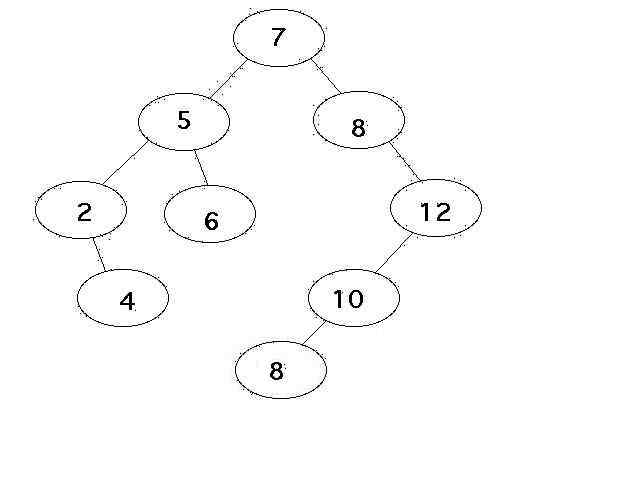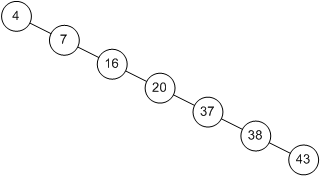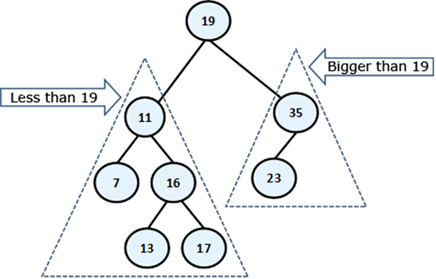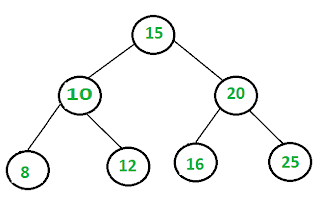Welcome to Lesson 10!

Learning Objectives
By the end of this class, you should know...
• How do you determine if a Binary Tree is balanced?
• How do you define a Binary Search Tree?
• How does a Binary Search Tree differ from a Binary Tree?
• What are the Big O Run-times of the BST Operations?
• How Do You Insert Data Into a BST?

Announcements
• Quiz today after the break
• Assignment 3 due tonight at midnight
• No Lab this Friday due to Presidents Day Holiday
• Also No Office Hours
• No Class on Monday due to Presidents Day Holiday
• Project Reports returned
• Can correct and resubmit on Wednesday
• Will release Assignment 4 on Binary Search Trees tonight
• You will have 2 weeks to complete it
• Good idea to start this weekend as you have no lab assignment

• Take a moment to familiarize yourself with this class.
• How is the node being represented?
• What kind of data does this BST hold?
• What are the private fields of the BST?

#ifndef BST_H_
#define BST_H_

#include <cstddef>
#include <string>

using namespace std;
template<typename bstdata>
class BST {
private:
struct Node {
bstdata data;
Node* left;
Node* right;

Node(bstdata newdata):left(NULL), right(NULL), data(newdata) {}
};

typedef struct Node* NodePtr;

NodePtr root;

/**Private helper functions*/
void insertData(NodePtr root, bstdata value);
//private helper function for insert
//recursively inserts a value into the BST

void inOrderPrint(ostream& out, NodePtr root);
//private helper function for inOrderPrint
//recursively prints tree values in order from smallest to largest

void preOrderPrint(ostream& out, NodePtr root);
//private helper function for preOrderPrint
//recursively prints tree values in pre order

void postOrderPrint(ostream& out, NodePtr root);
//private helper function for postOrderPrint
//recursively prints tree values in post order

void makeCopy(NodePtr copy);
//recursive helper function to the copy constructor

bool search(NodePtr root, bstdata value);
//recursive helper function to search
//returns whether the value is found in the tree

bstdata minimum(NodePtr root);
//recursive helper function to minimum
//returns the minimum value in the tree

bstdata maximum(NodePtr root);
//recursive helper function to maximum
//returns the maximum value in the tree

NodePtr deleteData(NodePtr root, bstdata value);
//recursive helper function to deleteData
//removes value from the tree

void size(NodePtr root, int& size);
//recursive helper function to the size function
//calculates the size of the tree
//stores the result in size

int height(NodePtr root);
//recursive helper function to the height function
//returns the height of the tree

public:

/**constructors and destructor*/

BST();
//Instantiates a new BST

BST(const BST &bst);
//copy constructor

~BST();
//deallocates the tree memory

/**access functions*/

bstdata minimum();
//returns the minimum value in the BST
//pre: !empty()

bstdata maximum();
//returns the maximum value in the BST and returns it
//pre: !empty()

bool empty();
//determines whether the BST is empty

int size();
//returns the size of the tree

bstdata getRoot();
//returns the value stored at the root of the BST
//pre: !empty()

int height();
//returns the height of the tree

bool search(bstdata value);
//returns whether the value is found in the tree
//pre: !empty()

/**manipulation procedures*/

void insertData(bstdata value);
//inserts a new value into the BST

void removeData(bstdata value);
//removes the specified value from the tree
//pre: value is located in the tree

void inOrderPrint(ostream& out);
//calls the inOrderPrint function to print out the values
//stored in the BST

void preOrderPrint(ostream& out);
//calls the reOrderPrint function to print out the values
//stored in the BST

void postOrderPrint(ostream& out);
//calls the postOrderPrint function to print out the values
//stored in the BST
};

#endif /* BST_H_ */
• Copy and paste the above into a new header file named BST.h
• Next, write the default constructor for this class.

BST Functions: InsertData

• As always, the best functions to write first are your default constructor, an insertion function and a print function.
• We have the default constructor, let's write our add function next.
• The functions you will be writing for the BST will take advantage of the recursive structure of the BST.
• Let's look at how this would work for insertion.
• Imagine that we are trying to insert 4 into our BST as in the image below.
• At each root node of a subtree, we need to make a decision. Do we go left? Do we go right? Or if the root node is null, do we insert right we should add right where we are.Image source.

• Thus, our process is recursive as we are making the same decision at each node.
• Our BST users do not have direct access to any of the nodes in the BST or their data or links.
• Therefore, we need to call a private helper function inside of insert and pass the root node to this function.
• The private helper function is the recursive function that is going to do most of the work here.
• The private helper function is called insert_value and it takes the root and a value as parameters.
• The public wrapper function is called insert and it simply takes a value.
• Here is the code for the public wrapper function:
template <class bstdata>
void BinarySearchTree<bstdata>::insertData(bstdata value)
{
if (root == NULL)
{
root = new Node(value); //if the tree is empty insert the value at the root
}
else
{
insertData(root, value); //otherwise call the helper function, passing it the root
}
}
• Here is the pseudocode for the private helper function:

Pseudocode for insertData(root, value)

1. Base Case: the value to insert is equal to the data stored at the root

If this is true, return //no duplicates

2. Check if the value to insert is smaller than the data stored at the root.

2a. If this is true

2aa. Check if root left is NULL

2aaa. If this is true, insert the value at root left

2b. If the root left is not NULL

2bb.recursively call the insertData function passing it root left and value

3. Otherwise

3a. Check if the root right is NULL

3aa. If this is true, insert the value at root right

3b. If the root right is not NULL

3bb. recursively call the insertData function passing it root right and value

Binary Tree Review

• What are the root and leaf nodes in the following tree?
• Is the Tree a binary tree? Why or Why Not?
• What are the depth and height of node 5? What are the depth and height of the root?Introduction to Run-time Analysis on Binary Trees
• The properties above are useful in analyzing the Big-O run-time of the binary tree operations.
• The run-time of binary tree operations is closely allied to the height of the tree.
• The closer the tree is to a perfect binary tree, the better the run-time.
• Why is this?
• Consider a binary tree like this one:• This binary tree is no different than a linked list.
• Is search efficient on a linked list? We should have seen in Lab 4 that searching a linked list is not efficient.
• Because linked lists do not offer random access of their elements, binary search is not an efficient algorithm on a linked list.
• Did you notice this inefficiency while completing your Lab 5?
• Thus, linear search is our better choice.
• What is the Big-O run-time of linear search on a linked list: O(n).
• For binary trees organized like the one above, search also takes O(n) time.
• We are going to see with balanced Binary Search Trees that search is possible in O (log n) time, just like binary search on an array.
• However, to keep search (and other operations) efficient, we want to keep our trees as balanced as possible.
• This idea should make intuitive sense to you.

Balanced Binary Tree
• But what does balanced mean?
• A balanced binary tree is one in which the difference between the height of the left and right subtrees is not more than 1.
| heightleft - heightright| <= 1
• Let's give this a try.
• The below tree is balanced even though it might not appear so. Why?• Consider the height of the right and left subtree of the root node A.
• hleft = 1
• hright = 2
• |1 - 2| = 1 <--balanced!
• Let's check another node, B.
• hleft = -1 (height of an empty tree is -1)
• hright = 0
• |-1 - 0| = 1 <--balanced!

Partner Activity
• With a partner answer the following question: Is the binary tree depicted below balanced? Why or why not?Image source.

• A Binary Search Tree (BST) is a binary tree for which the following is true: for any node in the BST, the value of all nodes in its left subtree are less than or equal to the value of the node, and the value at all nodes in the right subtree are greater than the value of the node.
• The following tree is a BST. Verify that the above property is true for this tree.BST: A Recursive Structure

• Remember that the root node is linked to the root nodes of its left and right subtrees.
• These root nodes are in turned linked to the root nodes of other subtrees.
• BST are recursive because the BST property must hold true for all nodes in the tree and their subtrees.• Examine the tree above. Do all subtrees meet the requirements for a BST?
• Due to the recursive definition of BSTs, we are able to implement most BST operations recursively, as we shall see in a moment.
Comparison of Arrays, Linked Lists and Binary Search Trees
• Why BSTs?
• Let's compare the average case Big-O run-times of Arrays and Linked Lists to those of the BST when performing 3 common ADT operations.
Array            Linked List            Binary Search Tree
Search                O(log n)                O(n)                    O(log n)
Insertion             O(n)                     O(n)                    O(log n)
Deletion              O(n)                     O(n)                    O(log n)

• Why are these operations O(log n) for a BST?
• Consider search. In the image below, we are searching for the value 4 to determine if it is in the tree:• As in binary search, we continually divide the search space in half until it is only one element:
n -> n / 2 -> n / 4 -> n/ 8... -> n/ 2k = 1
• Multiply both sides by 2k
n = 2k
• Then take the log of both sides:
log (n) = k
• This leaves us with O(log n).
• Let's walk through an example to verify this works on a balanced BST. What happens if we search for the value 12?• Insertion and removal have the same run-time on average as search. Let's walk through an example of inserting the value 9 in the BST above.
• But, what about when the BST is unbalanced?
• The Big-O run-time in this case will be equal to that of a linked list: O(n)
• Why?• We are going to build this header file piece-by-piece.
• Here is our bare-bones header file for the work we are going to do on our BST today.
• We will add public functions and private helper functions as we go along.
• Take a moment to familiarize yourself with this class.
• How is the node being represented?
• What kind of data does this BST hold?
• What are the private fields of the BST?

#ifndef BST_H_
#define BST_H_

#include <cstddef>
#include <string>

using namespace std;

template <class bstdata>
class BST
{
private:
struct Node
{
bstdata data;
Node* left;
Node* right;

Node(): left(NULL), right(NULL){}
Node(bstdata newdata): left(NULL), right(NULL), data(newdata){}
};

typedef struct Node* Nodeptr;

Nodeptr root;
int size;

/**Private helper functions*/

void printInOrder(Nodeptr root);

public:
BST();
bool isEmpty();
int getSize();
bstdata getRoot();
void inOrderPrint();

//more functions to be added here!

};

Activity 10.1 BST Functions: Default Constructor (10 pts)

• Create a new file called BST.h.
• Copy and paste the code above into your BST.h file.
• Then, write the default constructor for your BST below the definition of the BST class.
• When you are finished, submit your code to Catalyst.

• As always, the best functions to write first are your default constructor, an add function and a print function.
• We have the default constructor, let's write our add function next.
• The functions you will be writing for the BST will take advantage of the recursive structure of the BST.
• Let's look at how this would work for add.
• Imagine that we are trying to add 4 into our BST as in the image below.
• At each root node of a subtree, we need to make a decision. Do we go left? Do we go right? Or if the root node is null, do we insert right we should add right where we are.Image source.

• Thus, our process is recursive as we are making the same decision at each node.
• Because of information hiding, our BST users do not have direct access to any of the nodes in the BST or their data or links.
• Therefore, we need to call a private helper function inside of add and pass the root node to this function.
• The private helper function is the recursive function that is going to do most of the work here.
• The private helper function is called addValue and it takes the root and a value as parameters.
• The public wrapper function is called add and it simply takes a value.
• Here is the code for the public wrapper function:

template <class bstdata>
{
if (root == NULL)

{
root = new Node(value); //if the tree is empty insert the value at the root

size++;

}
else
addValue(root, value); //otherwise call the helper function, passing it the root

}

• Here is the pseudocode for the private helper function:

1. Base Case: the value to insert is equal to the data stored at the root

If this is true, return

2. Check if the value to insert is smaller than the data stored at the root.

2a. If this is true

2aa. Check if root left is NULL

2aaa. If this is true, insert the value at root left and adjust size

2b. If the root left is not NULL

2bb.recursively call the addValue function passing it root left and value

3. Otherwise

3a. Check if the root right is NULL

3aa. If this is true, insert the value at root right and adjust size

3b. If the root right is not NULL

3bb. recursively call the addValue function passing it root right and value

• Note that you will need to figure out where to add 1 to the size.